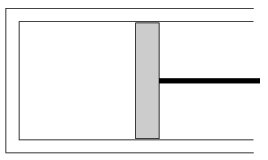Mathematical and Physical Journal
for High Schools
Issued by the MATFUND Foundation
 Already signed up? New to KöMaL?

#Problem P. 4130. (January 2009)

P. 4130. The horizontal cylinder shown in the figure and the piston in it, which can move without friction, are adiabatically insulated. The mass of the piston is 10 kg, the area of its cross-section is 50 cm2. The temperature of the air confined in the cylinder is 27 oC, its initial pressure is equal to the atmospheric pressure. The cylinder is slowly turned into the vertical position. By what value does its temperature change?(5 pont)

Deadline expired on February 11, 2009.

### Statistics:

 116 students sent a solution. 5 points: 94 students. 4 points: 6 students. 3 points: 6 students. 2 points: 5 students. 1 point: 3 students. Unfair, not evaluated: 2 solutionss.

Problems in Physics of KöMaL, January 2009